# SSAT Elementary Level Math : How to find the perimeter of a rectangle

## Example Questions

1 2 8 9 10 11 12 13 14 16 Next →

### Example Question #151 : How To Find The Perimeter Of A Rectangle

Use the following rectangle to solve the problem: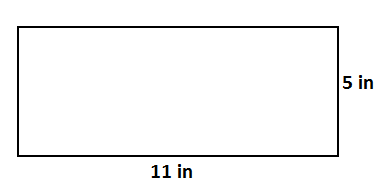Find the perimeter.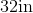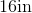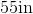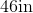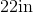Explanation:

To find the perimeter of a rectangle, we will use the following formula: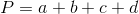Where a, b, c, and d are the lengths of the sides of the rectangle.

Now, given the rectanglewe can see the length is 11in.  Because it is a rectangle, the opposite side is also 11in.

The width is 5in.  Because it is a rectangle, the opposite side is also 5in.

So, we can substitute.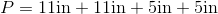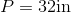### Example Question #51 : Geometry

What is the length of a room with a perimeter of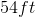and a width of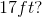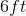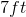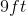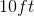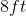Explanation: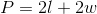We have the perimeter and the width, so we can plug those values into our equation and solve for our unknown.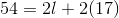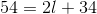Subtractfrom both sides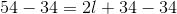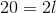Divideby both sides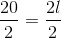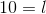1 2 8 9 10 11 12 13 14 16 Next →

### All SSAT Elementary Level Math Resources